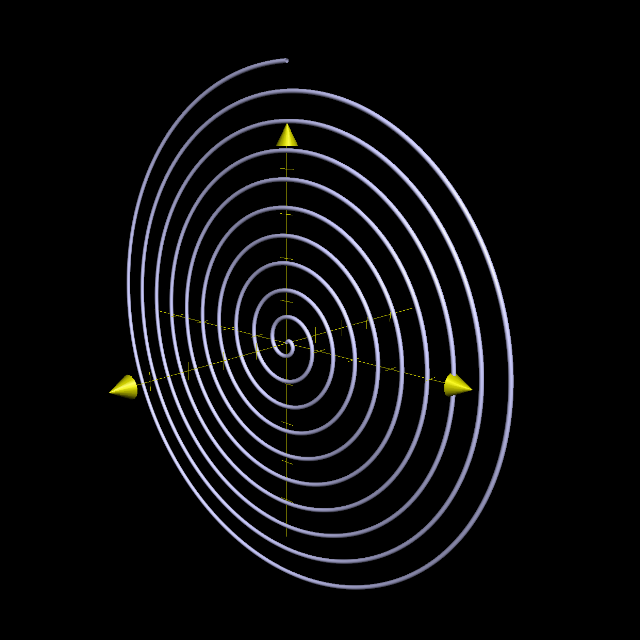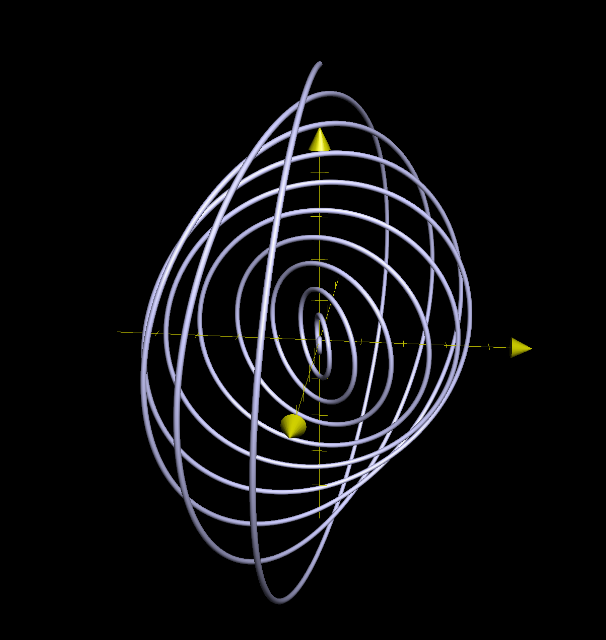## Wednesday, May 11, 2016

### Yitz..'s Kewel Spiral

Every once in a while, I get an "assignment" to calculate some interesting parametrization (because parametrizations are awesome like that). For example, the bowtie pasta was originally a request from a student. Today's goes way back, to someone asking a question on a forum in math way back when. He needed a spiral that started out and twisted its way through space as it got larger. In essence, a spiral that doesn't stay in the same plane. Obviously, polar curves are out of the question, since they are, well, in the plane. But what's one dimension up from polar coordinates? Spherical coordinates $(r,\varphi,\theta)$, of course (or if you're a physicist, $(r,\theta,\phi)$).We fall back on a good ol' tool, parametrization.

To discover what its equation might be, we first consider an Archimedean spiral ($r = a\varphi$) in the $r\varphi$-plane (or $yz$-plane), or possibly a logarithmic one, like $e^{\varphi/3}$, which grows as it goes around (like some spirals last time) the "pole" which is now the $x$-axis. This ends up growing as you move clockwise, rather than counterclockwise, since $\varphi$ increases as it goes from north toward east.

This is most efficiently represented in a parametric manner: first, in the $yz$-plane:$x=0,$ $y = at \sin t,$ $z = at \cos t.$
gives the parametric equation of the Archimedean spiral in the $yz$-plane going clockwise. This gives $\varphi = t$ and $r = at$, which recovers the original polar equation $r = a\varphi$.Archimedean Spiral in the $yz$-plane, with $a = 1/20$.

How do we give it a twist? We reason that, after each revolution, we want it twisted out of the plane by some small amount (say, rotated about the $z$-axis by $b$ times a full revolution): we apply the rotation to the $x$ and $y$, giving:$x=at\sin t \cos (bt),$ $y = at \sin t \sin(bt),$ $z = at \cos t.$Twisting out of the plane with parameter $b = 1/20$, also.

Here's the original one I delivered to Yitz.. (it is actually exponential):
Pretty kewel, right? (that was his exact word to describe it).

1 I always use the convention that if I indeed need to refer to a physics text, do calculations to be consistent... but I always write my $\phi$'s as non-curly in that context... I haven't yet figured how to draw my $\theta$s different though. Yes, I know there is $\vartheta$, but it hasn't caught on in my notation. It just doesn't feel enough like a $\theta$.

#### 1 comment:

1.stumbled upon this from google looking for spherical spirals with varying r. this is super cool and exactly what I was looking for, thanks for posting these!